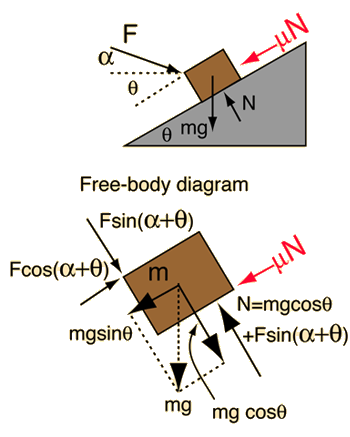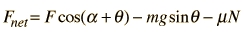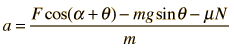# Force on Inclined MassThough describable by the motion equations and the standard model of friction, this example requires care because under different conditions the mass can move up or down the incline, or it can be just held in place by the applied force. In the diagrams above, it is assumed that the mass is moving up the incline, so that the frictional resistance acts downward to oppose it. If the mass is moving downward, then the frictional resistance force must be reversed in direction.
Application of Newton's second law to mass on incline.

A force of Newtons is applied at an angle of degrees above the horizontal to a mass of kg which sits on an incline of angle degrees. There is a coefficient of friction μ = between the mass and the incline. For a sufficient applied force so that the mass moves upward, the net force is given by the expressionand the resultant upward acceleration is given by### Acceleration =m/s2

The expressions above are valid only for the case where the applied force is sufficient to give an upward acceleration. If the applied force is too small to accelerate the mass upward, then there is a range of forces where the mass will not move, and if the incline is steep enough there is a range where the mass will accelerate downward. In the latter case, the friction force must be reversed in direction in the expression above since it will then act upward to oppose the downward motion. If the acceleration above is negative, that reversal was done in the calculation of the number. If the acceleration is zero,then it is in the range of conditions where the force will hold the mass on the incline, neither moving up nor down.

### Expressions****Remove friction

Index

Newton's laws

Standard mechanics problems

 HyperPhysics***** Mechanics R Nave
Go Back